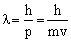# Chemistry Assignment Help with Radial Probability Distribution Curves

1. Home
2. Chemistry
3. Atomic Structure

## 3.15 Radial Probability Distribution Curves

The maximum in the curve indicates the most probable value and the corresponding distance from the nucleus is called distance of maximum probability. For hydrogen atom, this radius has a value of 53 pm. The radial probability distribution curve for 2s orbital shows two maxima, a smaller one near the nucleus and bigger one at a larger distance. In between two maxima, there is one minima where there is no probability of finding the electron (nodal point). The distance of maximum probability for 2p and 2s orbitals are same and this distance is greater than that of 1s orbital. Hence 1s orbital is nearer to the nucleus in comparison to 2s and 2p orbitals.The radius of maximum probability of 2s orbital is greater than 2p orbital. In the case of 2s orbital, one additional maxima appears at small distance, this confirms that the 2s electrons spends more time near the nucleus than 2p electrons. Hence, 2s orbital is more penetrating than 2p orbital.### Email Based Assignment Help in Atomic Structure

Radial Probability Distribution Curves is a topic in chemistry atomic structure. If you want Assignment Help homework help in Radial Probability Distribution Curves,
assignmenthelp.net online tutors is available for you.